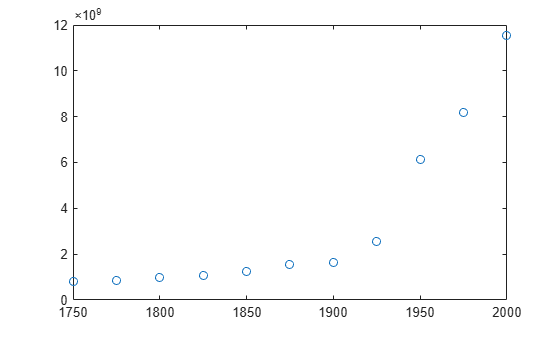# polyval

## 语法

``y = polyval(p,x)``
``[y,delta] = polyval(p,x,S)``
``y = polyval(p,x,[],mu)``
``[y,delta] = polyval(p,x,S,mu)``

## 说明

``y = polyval(p,x)` 计算多项式 `p` 在 `x` 的每个点处的值。参数 `p` 是长度为 `n+1` 的向量，其元素是 `n` 次多项式的系数（降幂排序）：$p\left(x\right)={p}_{1}{x}^{n}+{p}_{2}{x}^{n-1}+...+{p}_{n}x+{p}_{n+1}.$虽然可以为不同目的使用 `polyint`、`polyder` 和 `polyfit` 等函数计算 `p` 中的多项式系数，但您也可以为系数指定任何向量。要以矩阵方式计算多项式，请改用 `polyvalm`。`

``[y,delta] = polyval(p,x,S)` 使用 `polyfit` 生成的可选输出结构体 `S` 来生成误差估计值。`delta` 是使用 `p(x)` 预测 `x` 处的未来观测值时的标准误差估计值。`

``y = polyval(p,x,[],mu)` 或 `[y,delta] = polyval(p,x,S,mu)` 使用 `polyfit` 生成的可选输出 `mu` 来中心化和缩放数据。`mu(1)` 为 `mean(x)`，`mu(2)` 为 `std(x)`。使用这些值时，`polyval` 将 `x` 的中心置于零值处并缩放为具有单位标准差$\stackrel{^}{x}=\frac{x-\overline{x}}{{\sigma }_{x}}\text{\hspace{0.17em}}.$这种中心化和缩放变换可改善多项式的数值属性。`

## 示例

```p = [3 2 1]; x = [5 7 9]; y = polyval(p,x)```
```y = 1×3 86 162 262 ```

`$I={\int }_{-1}^{3}\left(3{x}^{4}-4{x}^{2}+10x-25\right)dx.$`

`p = [3 0 -4 10 -25];`

`q = polyint(p)`
```q = 1×6 0.6000 0 -1.3333 5.0000 -25.0000 0 ```

```a = -1; b = 3; I = diff(polyval(q,[a b]))```
```I = 49.0667 ```

```x = 1:100; y = -0.3*x + 2*randn(1,100); [p,S] = polyfit(x,y,1); ```

`[y_fit,delta] = polyval(p,x,S);`

```plot(x,y,'bo') hold on plot(x,y_fit,'r-') plot(x,y_fit+2*delta,'m--',x,y_fit-2*delta,'m--') title('Linear Fit of Data with 95% Prediction Interval') legend('Data','Linear Fit','95% Prediction Interval')``````year = (1750:25:2000)'; pop = 1e6*[791 856 978 1050 1262 1544 1650 2532 6122 8170 11560]'; T = table(year, pop)```
```T=11×2 table year pop ____ _________ 1750 7.91e+08 1775 8.56e+08 1800 9.78e+08 1825 1.05e+09 1850 1.262e+09 1875 1.544e+09 1900 1.65e+09 1925 2.532e+09 1950 6.122e+09 1975 8.17e+09 2000 1.156e+10 ```
`plot(year,pop,'o')``[p,~,mu] = polyfit(T.year, T.pop, 5);`

```f = polyval(p,year,[],mu); hold on plot(year,f) hold off```## 输入参数

`R`Vandermonde 矩阵 `x` 的 QR 分解的三角因子
`df`自由度
`normr`残差的范数